# Search Results (11)

View
Selected filters:
• CCSS.Math.Content.8.EE.A.3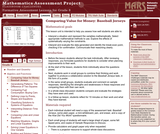Rating

This lesson unit is intended to help teachers assess how well students are able to: interpret a situation and represent the variables mathematically; select appropriate mathematical methods to use; explore the effects of systematically varying the constraints; interpret and evaluate the data generated and identify the break-even point, checking it for confirmation; and communicate their reasoning clearly.

Material Type:
Assessment
Lesson Plan
Provider:
Shell Center for Mathematical Education
U.C. Berkeley
Provider Set:
Mathematics Assessment Project (MAP)
Mathematics Assessment Project (MAP)
04/26/2013Rating

This lesson unit is intended to help teahcers assess how well students are able to interpret speed as the slope of a linear graph and translate between the equation of a line and its graphical representation.

Material Type:
Assessment
Lesson Plan
Provider:
Shell Center for Mathematical Education
U.C. Berkeley
Provider Set:
Mathematics Assessment Project (MAP)
Mathematics Assessment Project (MAP)
04/26/2013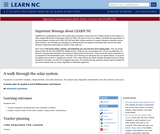Conditions of Use:
Remix and Share
Rating

A practice in scientific notation, measurement, and scale distances, this lesson plan integrates mathematics into the science curriculum.

Material Type:
Lesson Plan
Provider:
University of North Carolina at Chapel Hill School of Education
Provider Set:
LEARN NC Lesson Plans
Author:
RhondaS Dausman
06/25/1999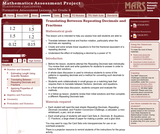Rating

This lesson unit is intended to help teachers assess how well students are able to: translate between decimal and fraction notation, particularly when the decimals are repeating; create and solve simple linear equations to find the fractional equivalent of a repeating decimal; and understand the effect of multiplying a decimal by a power of 10.

Material Type:
Assessment
Lesson Plan
Provider:
Shell Center for Mathematical Education
U.C. Berkeley
Provider Set:
Mathematics Assessment Project (MAP)
Mathematics Assessment Project (MAP)
04/26/2013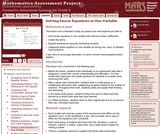Rating

This lesson unit is intended to help teachers assess how well students are able to: solve linear equations in one variable with rational number coefficients; collect like terms; expand expressions using the distributive property; and categorize linear equations in one variable as having one, none, or infinitely many solutions. It also aims to encourage discussion on some common misconceptions about algebra.

Material Type:
Assessment
Lesson Plan
Provider:
Shell Center for Mathematical Education
U.C. Berkeley
Provider Set:
Mathematics Assessment Project (MAP)
Mathematics Assessment Project (MAP)
04/26/2013Rating

This lesson unit is intended to help teachers assess how well students are able to classify solutions to a pair of linear equations by considering their graphical representations. In particular, this unit aims to help teachers identify and assist students who have difficulties in: using substitution to complete a table of values for a linear equation; identifying a linear equation from a given table of values; and graphing and solving linear equations.

نوع المادة:
التقييم
Lesson Plan
Provider:
Shell Center for Mathematical Education
U.C. Berkeley
Provider Set:
Mathematics Assessment Project (MAP)
Mathematics Assessment Project (MAP)
04/26/2013Rating

يهدف هذا الدرس لمساعدة المعلمين على تقييم مدى نجاح الطلاب في إنشاء المعادلات الخطية وحلها. على وجه الخصوص، يساعدك الدرس على تحديد الطلاب الذين لديهم الصعوبات الآتية ومساعدتهم: حل عادلات بمتغير واحد وحل المعادلات الخطية بأكثر من طريقة واحدة.

نوع المادة:
التقييم
Lesson Plan
Provider:
Shell Center for Mathematical Education
U.C. Berkeley
Provider Set:
Mathematics Assessment Project (MAP)
Mathematics Assessment Project (MAP)
04/26/2013Rating

This lesson unit is intended to help you assess how well students working with square numbers are able to: choose an appropriate, systematic way to collect and organize data, examining the data for patterns; describe and explain findings clearly and effectively; generalize using numerical, geometrical, graphical and/or algebraic structure; and explain why certain results are possible/impossible, moving towards a proof.

Material Type:
Assessment
Lesson Plan
Provider:
Shell Center for Mathematical Education
U.C. Berkeley
Provider Set:
Mathematics Assessment Project (MAP)
Mathematics Assessment Project (MAP)
04/26/2013Conditions of Use:
No Strings Attached
Rating

This module contains the basic operations with real numbers from Elementary Algebra by Denny Burzynski and Wade Ellis, Jr.

Material Type:
Provider:
Rice University
Provider Set:
Connexions
Author:
Denny Burzynski
02/16/2011Rating

This lesson unit is intended to help teachers assess how well students are able to: estimate lengths of everyday objects; convert between decimal and scientific notation; and make comparisons of the size of numbers expressed in both decimal and scientific notation.

Material Type:
Assessment
Lesson Plan
Provider:
Shell Center for Mathematical Education
U.C. Berkeley
Provider Set:
Mathematics Assessment Project (MAP)
Mathematics Assessment Project (MAP)
04/26/2013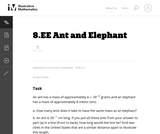Conditions of Use:
No Strings Attached
Rating

In this problem students are comparing a very small quantity with a very large quantity using the metric system. The metric system is especially convenient when comparing measurements using scientific notations since different units within the system are related by powers of ten.

Material Type:
Activity/Lab
Provider:
Illustrative Mathematics
Provider Set:
Illustrative Mathematics
Author:
Illustrative Mathematics